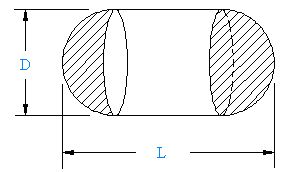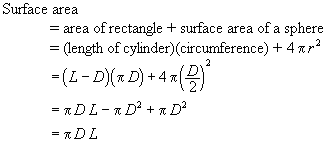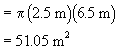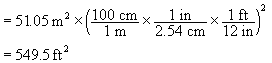# Petroleum: Determining the Surface Area of an Oval Tank.1. Find and simplify a formula for the surface area of the oval tank shown to the right.
2. If the diameter is D = 2.5 meters and the length is L = 6.5 meters then what is the surface area in square feet?

## Solution:

1. The formula for the surface area of the tank is:We have discovered that the area is equal to the area of an open cylinder L units long (one with no ends).

2. The surface area in square meters isThe surface area in square feet isWritten by Graham Cocksedge, September 21, 1997